# NCERT Solutions for Introduction to Graphs Chapter 15 Class 8 Mathematics CBSE Exercise 15.2

In this page we have NCERT book Solutions for Class 8th Maths:Introduction to Graphs Chapter 15 for Exercise 15.2 . Hope you like them and do not forget to like , social share and comment at the end of the page.
Question 1
Plot the following points on a graph sheet. Verify if they lie on a line
(a) A(4, 0), B(4, 2), C(4, 6), D(4, 2.5)
(b) P(1, 1), Q(2, 2), R(3, 3), S(4, 4)
(c) K(2, 3), L(5, 3), M(5, 5), N(2, 5)
a)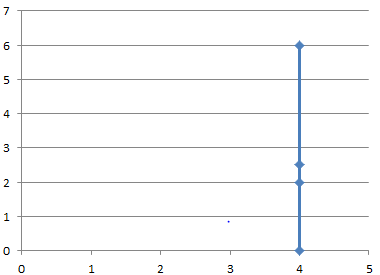As we can see, all the points lie on the straight line
b)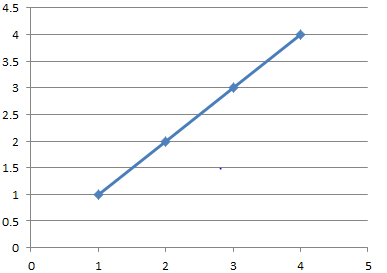As we can see, all the points lie on the straight line
c)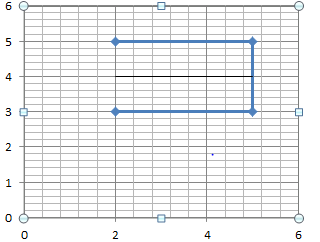All points are not in straight line
Question 2
Draw the line   passing through the points (2,3)  and (3,2) and find the coordinate of the points at which it meets x –axis and y –axis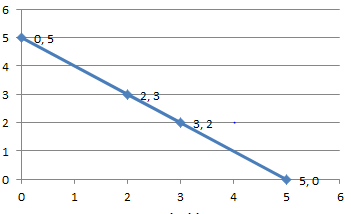The coordinates of the points where intersect xaxis and yaxis are 0,5 and 5,0

Question 3
Find the coordinates of each points in the below graph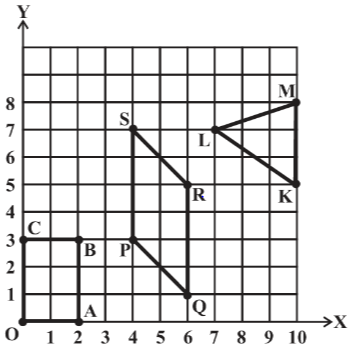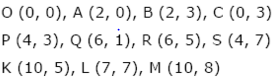Question 4
State whether True or False. Correct those are false.
(i) A point whose x coordinate is zero and y-coordinate is non-zero will lie on the y-axis.
(ii) A point whose y coordinate is zero and x-coordinate is 5 will lie on y-axis.
(iii) The coordinates of the origin are (0, 0).
i) True
ii) False
iii) True

Reference Books for class 8 Math

Given below are the links of some of the reference books for class 8 Math.

1. Mathematics Foundation Course for JEE/Olympiad : Class 8 This book can take students maths skills further. Only buy if child is interested in Olympiad/JEE foundation courses.
2. Mathematics for Class 8 by R S Aggarwal Detailed Mathematics book to clear basics and concepts. I would say it is a must have book for class 8 student.
3. Pearson Foundation Series (IIT -JEE / NEET) Physics, Chemistry, Maths & Biology for Class 8 (Main Books) | PCMB Combo : These set of books could help your child if he aims to get extra knowledge of science and maths. These would be helpful if child wants to prepare for competitive exams like JEE/NEET. Only buy if you can provide help to the child while studying.
4. Reasoning Olympiad Workbook - Class 8 :- Reasoning helps sharpen the mind of child. I would recommend students practicing reasoning even though they are not appearing for Olympiad.

You can use above books for extra knowledge and practicing different questions.

Note to our visitors :-

Thanks for visiting our website.